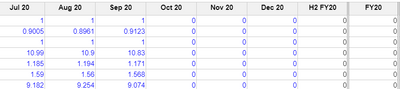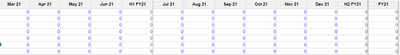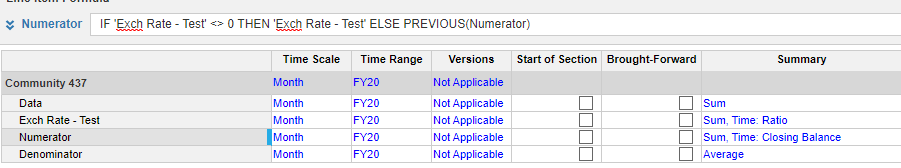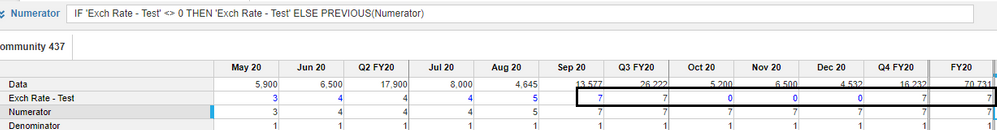# Retrieving last non-zero valueHi,

I created a module with exchange rates data for each month. Then, I put summary as : sum, time: closing balance.

But as I only have data until Sep 2020, it puts 0 for FY20 and 21. So, I would like to set up a formula that retrieve the last non-zero value for FY20 and FY21 for instance.Tagged:

•Unfortunately there is no LASTNONZERO function available, we will go for a workaround here. See if this helps

Step 1: Create a line item call it Numerator. Write below formula in it

IF 'Exch Rate - Test' <> 0 THEN 'Exch Rate - Test' ELSE PREVIOUS(Numerator) and change the summary to Sum, Closing Bal

Step 2: Create another line item call it Denominator.

Hardcode number 1 in  it and change the summary to Average

Step 3: Go to your target line item ( in my case Exch Rate - Test) and change the summary from Closing Balance to Ratio (Numerator/Denominator)